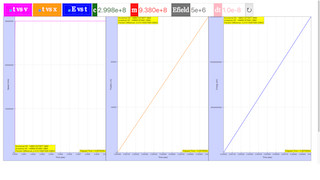Intro Page

Relativistic Dynamics in 1D with a constant force

Developed by Larry Engelhardt

In these exercises, you will determine the motion of a proton in a uniform electric field. We will begin by simulating a proton in an electric field using the NON-relativistic version of Newton’s 2nd Law. Then we will modify this simulation to take special relativity into account. In the process, we will observe the transition from non-relativistic to relativistic dynamics. In order to generate results, we will see that we need to be careful when working with non-SI units. In particular, we will need to pay close attention to factors of eV and $c$.

 Subject Area Modern Physics Beyond the First Year IPython/Jupyter Notebook and Python Students will be able to: Execute a working simulation, and explain non-relativistic, constant force motion (Exercise 1) Manipulate and explain the units that appear in the context of relativistic motion (Exercise 2) Interpret plots of energy vs. time for non-relativistic motion (Exercises 2) Observe and explain when the non-relativistic form of Newton’s 2nd Law breaks down (Exercise 3) Derive the relativistic form of Newton’s 2nd Law (Exercise 4) Modify a non-relativistic simulation to incorporate relativity (Exercise 5) Produce and interpret plots for relativistic motion (Exercises 6, 7, 8) Validate numerical results by comparing with an analytical solution (Exercise 7) Apply simulated results to a particle accelerator (Exercises 9 and 10) Rewrite code to store data in arrays using array indices rather than by appending data to arrays (Exercise 11) 120 min
Exercise 10

Exercise 10:

At the LHC, protons are accelerated using much larger electric fields, $ϵ=5×{10}^{6}$ Volts/meter. Use your program to simulate a proton at the LHC being accelerated by this large electric field. (Tip: You will need to significantly change the values of both the time step and the maximum time.) Discuss your results.

Exercise 11

Exercise 11 (RELEVANT FOR PYTHON OR MATLAB, NOT RELEVANT FOR ALL PROGRAMMING LANGAUGES):

You might have noticed that your program becomes very slow if you have a very large number of time steps. Part of this is unavoidable; each time step requires the computer to do some processessing. However, much of the computation time is consumed by appending new values onto the arrays.

Rewrite your program so that, instead of repeatedly appending to the arrays, you create large empty arrays before the loop; then in each iteration of the loop, you record the new numbers in the appropriate element of the array. Observe and discuss how this affects the computation time.

Complete_RelativisticDynamics_Faster.py

# relativisticDynamicsVersion3.py

from pylab import *

from time import time

start = time()

c = 2.998e8 # Speed of light in m/s

m = 0.938e9 # Mass in eV/c^2

Efield = 5e6 # Electric field in Volts per meter

x = 0 # Position in meters

v = 0 # Velocity in meters/second

t = 0 # Time in seconds

dt = 1e-8 # Time STEP in seconds

tMax = 1e-3

N = int(tMax / dt)

tArray = zeros(N)

xArray = zeros(N)

vArray = zeros(N)

EArray = zeros(N)

lorentzArray = zeros(N)

for i in range(N):

# The dynamics:

lorentz = 1 / sqrt(1 - v**2 / c**2)

a = Efield*c**2/(lorentz**3*m)

t = t + dt

x = x + v*dt

v = v + a*dt

E = lorentz * m

# Write the new values into the arrays

tArray[i] = t

xArray[i] = x

vArray[i] = v

EArray[i] = E

lorentzArray[i] = lorentz

end = time()

print('Elapsed time:', end-start)

# The following lines are added for Exercise 7

KE_numerical = lorentz * m - m

KE_analytical = Efield*x

print('Numerical KE: ', KE_numerical)

print('Analytical KE:', KE_analytical)

print('Percent Difference:', 100*abs(KE_numerical-KE_analytical)/KE_analytical)

# Create plots

figure(1)

plot(tArray, vArray, linewidth=2)

xlabel('Time (sec)')

ylabel('Speed (m/s)')

grid(True)

ylim([0, 3.1e8])

savefig('ultrarelativistic_v_vs_t.png')

show()

figure(2)

plot(tArray, xArray, linewidth=2)

xlabel('Time (sec)')

ylabel('Position (m)')

grid(True)

savefig('ultrarelativistic_x_vs_t.png')

show()

figure(3)

plot(tArray, EArray, linewidth=2)

xlabel('Time (sec)')

ylabel('Energy (eV)')

grid(True)

savefig('ultrarelativistic_E_vs_t.png')

show()

### Translations

Code Language Translator Run### Software Requirements

SoftwareRequirements

 Android iOS Windows MacOS with best with Chrome Chrome Chrome Chrome support full-screen? Yes. Chrome/Opera No. Firefox/ Samsung Internet Not yet Yes Yes cannot work on some mobile browser that don't understand JavaScript such as..... cannot work on Internet Explorer 9 and below

### CreditsFremont Teng; Loo Kang Wee; based on codes by Larry Engelhardt